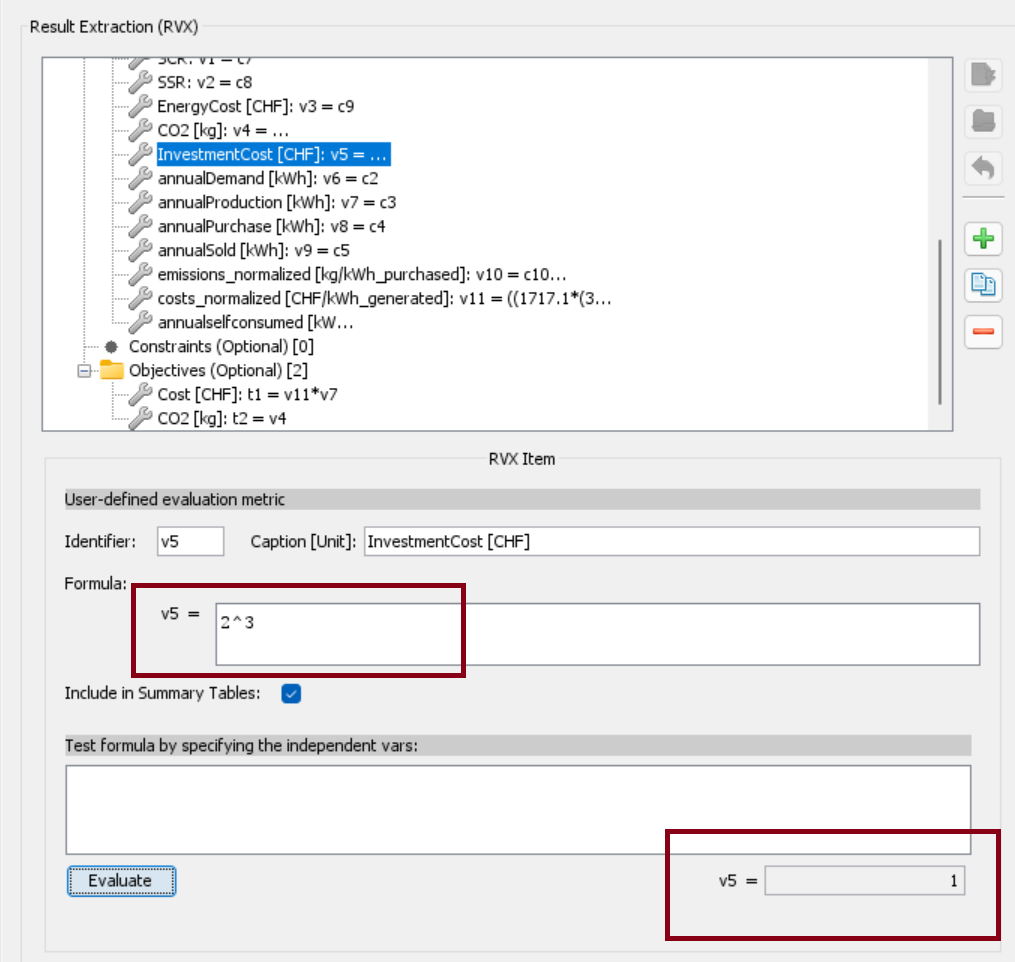Question-and-Answer Resource for the Building Energy Modeling Community
Get started with the Help page

# jEPlus: formula calculation error

Hi,

I am using jEPlus for optimization, and I used a formula to define the optimization objection.

I found the obtained results quite strange and I went back to check if I made a mistake in defining the formula,

and here I found out that the formula didn't work out for a to the power of b functions, I used the tested formula: v5=2^3 and the evaluation results calculated by jEPlus is 1 instead of 8, see the attached screenshot.

I was wondering if this is a bug for the calculation of this type of functions or if I defined it in a wrong way.

Thanks in advance.edit retag close merge delete

update: I tried the following formulas and the evaluation results are all wrong: v5 = 2^6 = 4; v5 = 2^7 = 5; v5 = 2^8 = 10; v5 = 2^9 = 11;

seems like jEPlus see "^" as either "+" or ''-''

Sort by » oldest newest most voted

problem solved: v5 should be defined as v5 = Math.pow(2, 3) instead of v5 = 2^3

more